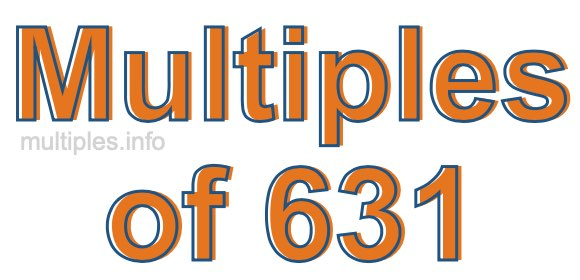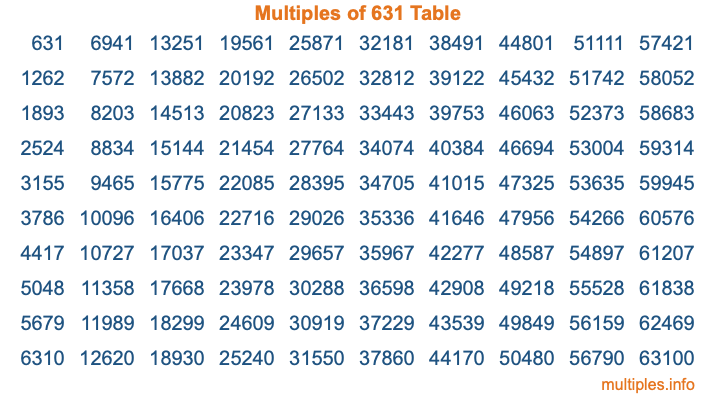Multiples of 631Welcome to the Multiples of 631 page. Here we will first teach you everything you will ever need to know about the multiples of 631, and then give you a study guide summary of everything we taught you to make sure you remember it all. Use this page to look up facts and learn information about the multiples of 631. This page will make you a multiples of six hundred thirty-one expert!

Definition of Multiples of 631
Multiples of 631 are all the numbers that when divided by 631 equal an integer. Each of the multiples of 631 are called a multiple. A multiple of 631 is created by multiplying 631 by an integer.

Therefore, to create a list of multiples of 631, you start with 1 multiplied by 631, then 2 multiplied by 631, then 3 multiplied by 631, and so on for as long as you want. Thus, the list of the first five multiples of 631 is 631, 1262, 1893, 2524, and 3155. To see a larger list of multiples of 631, see the printable image of Multiples of 631 further down on this page. We also have a category where you can choose any nth multiple of 631.

Multiples of 631 Checker
The Multiples of 631 Checker below checks to see if any number of your choice is a multiple of 631. In other words, it checks to see if there is any number (integer) that when multiplied by 631 will equal your number. To do that, we divide your number by 631. If the the quotient is an integer, then your number is a multiple of 631.

Is  a multiple of 631?

Least Common Multiple of 631 and ...
A Least Common Multiple (LCM) is the lowest multiple that two or more numbers have in common. This is also called the smallest common multiple or lowest common multiple and is useful to know when you are adding our subtracting fractions. Enter one or more numbers below (631 is already entered) to find the LCM.

Check out our LCM Calculator if you need more details about the Least Common Multiple or if you need the LCM for different numbers for adding and subtraction fractions.

nth Multiple of 631
As we stated above, 631 is the first multiple of 631, 1262 is the second multiple of 631, 1893 is the third multiple of 631, and so on. Enter a number below to find the nth multiple of 631.

th multiple of 631

Multiples of 631 vs Factors of 631
631 is a multiple of 631 and a factor of 631, but that is where the similarities end. All postive multiples of 631 are 631 or greater than 631. All positive factors of 631 are 631 or less than 631.

Below is the beginning list of multiples of 631 and the factors of 631 so you can compare:

Multiples of 631: 631, 1262, 1893, 2524, 3155, etc.

Factors of 631: 1, 631

As you can see, the multiples of 631 are all the numbers that you can divide by 631 to get a whole number. The factors of 631, on the other hand, are all the whole numbers that you can multiply by another whole number to get 631.

It's also interesting to note that if a number (x) is a factor of 631, then 631 will also be a multiple of that number (x).

Multiples of 631 vs Divisors of 631
The divisors of 631 are all the integers that 631 can be divided by evenly. Below is a list of the divisors of 631.

Divisors of 631: 1, 631

The interesting thing to note here is that if you take any multiple of 631 and divide it by a divisor of 631, you will see that the quotient is an integer.

Multiples of 631 Table
Below is an image of the first 100 multiples of 631 in a table. The table is in chronological order, column by column. The first column has the first ten multiples of 631, the second column has the next ten multiples of 631, and so on.The Multiples of 631 Table is also referred to as the 631 Times Table or Times Table of 631. You are welcome to print out our table for your studies.

Negative Multiples of 631
Although not often discussed or needed in math, it is worth mentioning that you can make a list of negative multiples of 631 by multiplying 631 by -1, then by -2, then by -3, and so on, to get the following list of negative multiples of 631:

-631, -1262, -1893, -2524, -3155, etc.

Multiples of 631 Summary
Below is a summary of important Multiples of 631 facts that we have discussed on this page. To retain the knowledge on this page, we recommend that you read through the summary and explain to yourself or a study partner why they hold true.

There are an infinite number of multiples of 631.

A multiple of 631 divided by 631 will equal a whole number.

631 divided by a factor of 631 equals a divisor of 631.

The nth multiple of 631 is n times 631.

The largest factor of 631 is equal to the first positive multiple of 631.

631 is a multiple of every factor of 631.

631 is a multiple of 631.

A multiple of 631 divided by a divisor of 631 equals an integer.

631 divided by a divisor of 631 equals a factor of 631.

Any integer times 631 will equal a multiple of 631.

Multiples of a Number
Here you can get the multiples of another number, all with the same attention to detail as we did for multiples of 631 on this page.

Multiples of
Multiples of 632
Did you find our page about multiples of six hundred thirty-one educational? Do you want more knowledge? Check out the multiples of the next number on our list!